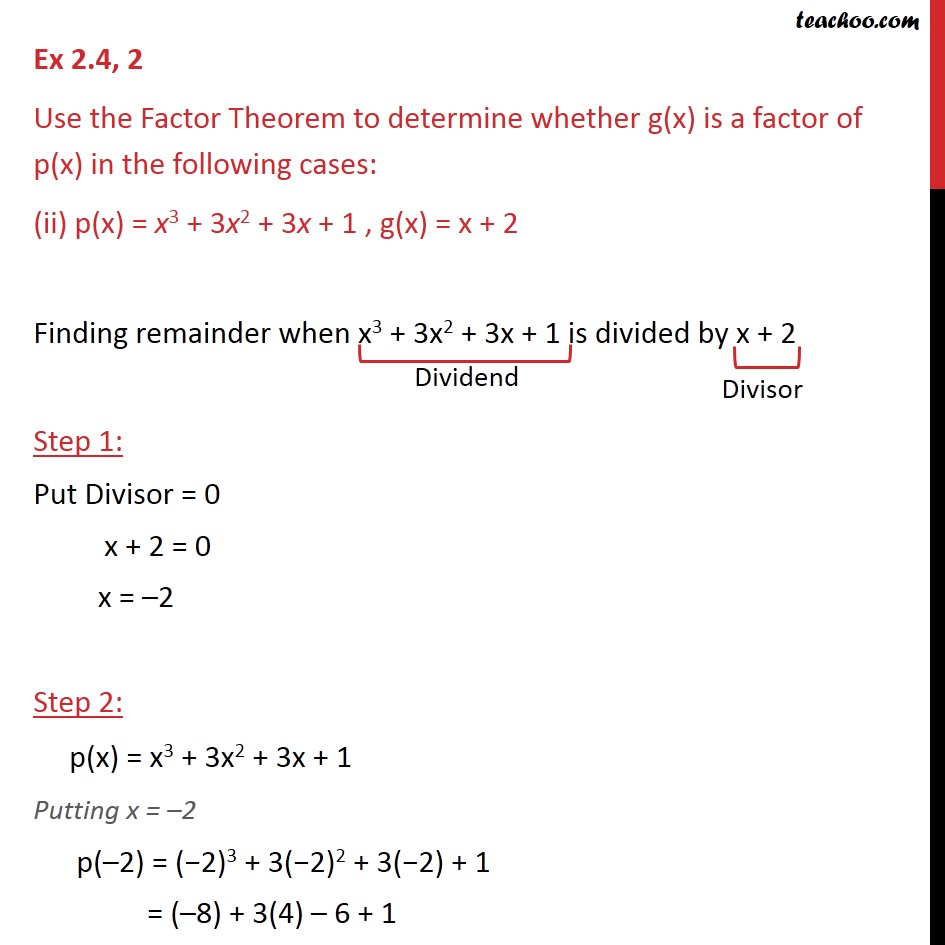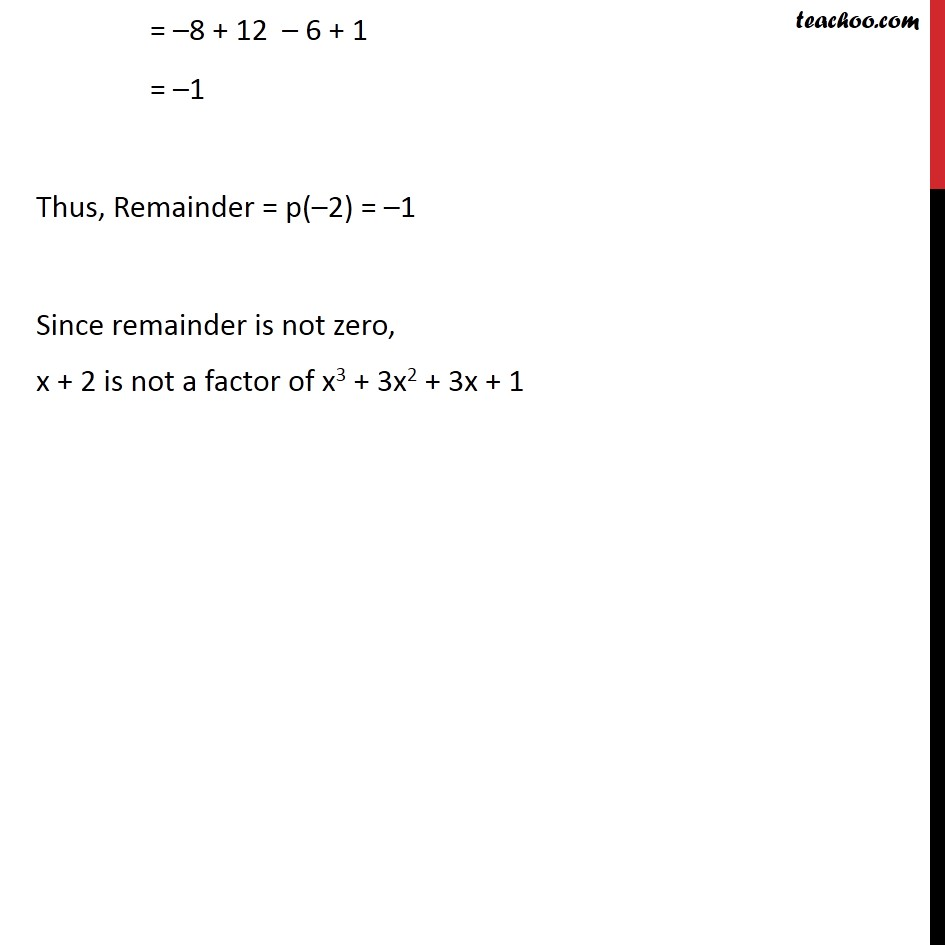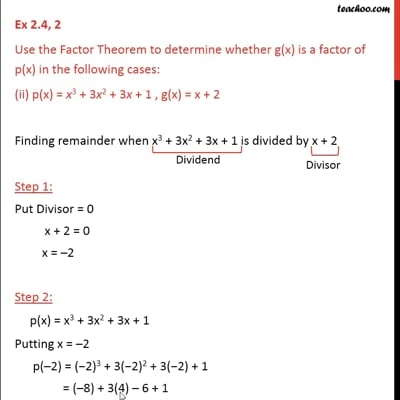Ex 2.3

Chapter 2 Class 9 Polynomials
Serial order wiseThis video is only available for Teachoo black users

Learn in your speed, with individual attention - Teachoo Maths 1-on-1 Class

### Transcript

Ex 2.3, 2 Use the Factor Theorem to determine whether g(x) is a factor of p(x) in the following cases: (ii) p(x) = x3 + 3x2 + 3x + 1 , g(x) = x + 2 Finding remainder when x3 + 3x2 + 3x + 1 is divided by x + 2 Step 1: Put Divisor = 0 x + 2 = 0 x = –2 Step 2: p(x) = x3 + 3x2 + 3x + 1 Putting x = –2 p(–2) = (−2)3 + 3(−2)2 + 3(−2) + 1 = (–8) + 3(4) – 6 + 1 = –8 + 12 – 6 + 1 = –1 Thus, Remainder = p(–2) = –1 Since remainder is not zero, x + 2 is not a factor of x3 + 3x2 + 3x + 1